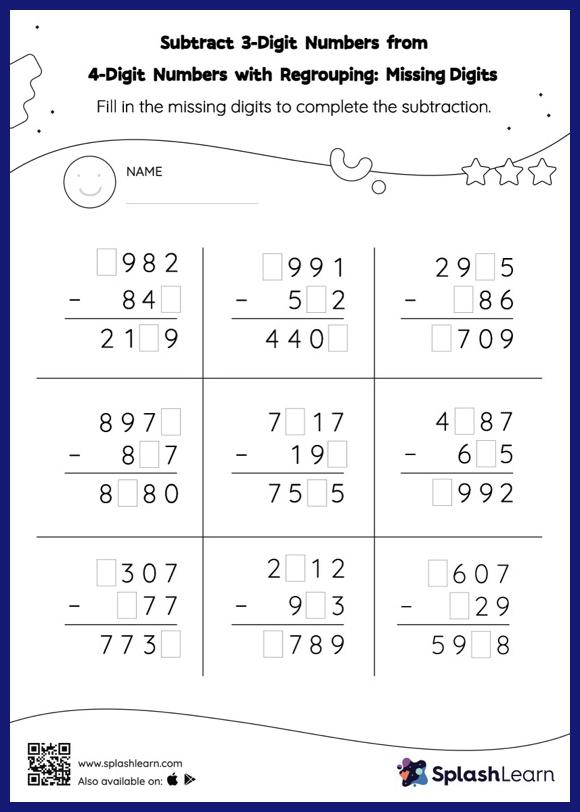# Subtract 3-Digit Numbers from 4-Digit Numbers with Regrouping: Missing Digits Worksheet

Home > Subtract 3-Digit Numbers from 4-Digit Numbers with Regrouping: Missing DigitsChildren practice subtracting 3-digit numbers from 4-digit numbers with regrouping with this worksheet. In this worksheet, students must find the missing number using the count back method or the relationship between addition and subtraction. To solve subtract 3-digit numbers from 4-digit numbers with regrouping worksheet, they must also regroup the numbers.